## 10.6.2 Motion planning with nature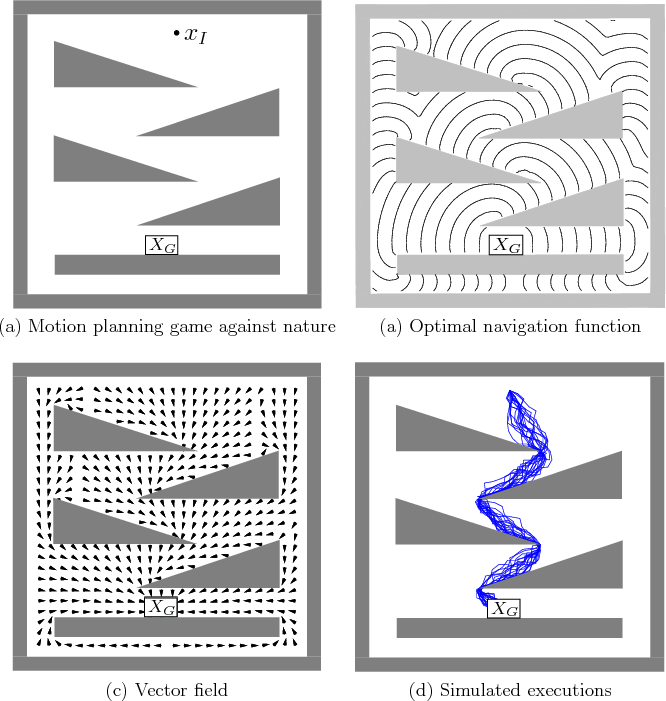Recall from Section 8.5.2 that value iteration with interpolation can be applied to motion planning problems that are approximated in discrete time. Nature can even be introduced into the discrete-time approximation. For example, (8.62) can be replaced by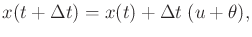(10.123)

in which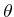is chosen from a bounded set,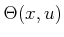. Using (10.124), value iterations can be performed as described so far. An example of a 2D motion planning problem under this model using probabilistic uncertainty is shown in Figure 10.20. It is interesting that when the plan is executed from a fixed initial state, a different trajectory is obtained each time. The average cost over multiple executions, however, is close to the expected optimum.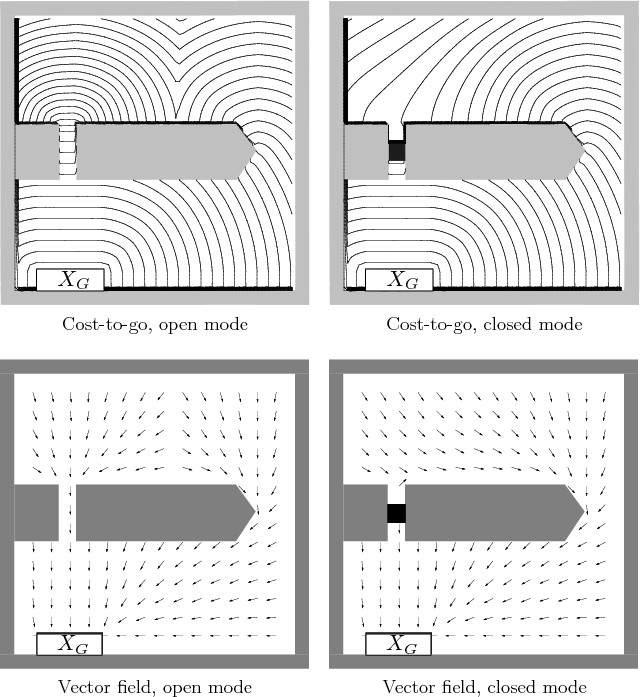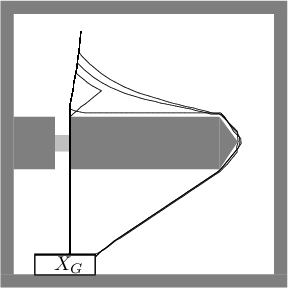Interesting hybrid system examples can be made in which nature is only allowed to interfere with the mode. Recall Formulation 7.3 from Section 7.3. Nature can be added to yield the following formulation.

Formulation 10..5 (Hybrid System Motion Planning with Nature)
1. Assume all of the definitions from Formulation 7.3, except for the transition functions,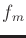and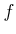. The state is represented as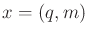.
2. A finite nature action spacefor each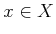and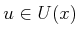.
3. A mode transition functionthat produces a mode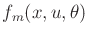for every,, and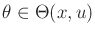.
4. A state transition functionthat is derived fromby changing the mode and holding the configuration fixed. Thus,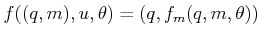(the only difference with respect to Formulation 7.3 is thathas been included).
5. An unbounded time interval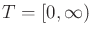.
6. A continuous-time cost-functional,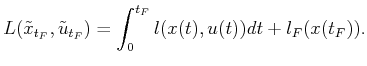(10.124)

Value iteration proceeds in the same way for such hybrid problems. Interpolation only needs to be performed over the configuration space. Along the mode axis'' no interpolation is needed because the mode set is already finite. The resulting computation time grows linearly in the number of modes. A 2D motion planning example for a point robot, taken from , is shown in Figures 10.21 and 10.22. In this case, the environment contains a door that is modeled as a stationary Markov process. The configuration space is sampled using a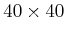grid. There are two modes: door open or door closed. Thus, the configuration space has two layers, one for each mode. The robot wishes to minimize the expected time to reach the goal. The navigation function for each layer cannot be computed independently because each takes into account the transition probabilities for the mode. For example, if the door is almost always open, then its plan would be different from one in which the door is almost always closed. If the door is almost always open, then the robot should go toward the door, even if it is currently closed, because it is highly likely that it will open soon. Numerous variations can be made on this example. More modes could be added, and other interpretations are possible, such as hazardous regions and shelters (the mode might be imagined as rain occurring and the robot must run for shelter) or requests to deliver objects [613,870,871].

Steven M LaValle 2020-08-14